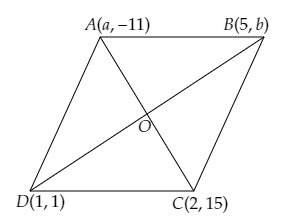# If the points $A (a, -11), B (5, b), C (2, 15)$ and $D (1, 1)$ are the vertices of a parallelogram $ABCD$, find the values of $a$ and $b$.

Given:

The points $A (a, -11), B (5, b), C (2, 15)$ and $D (1, 1)$ are the vertices of a parallelogram $ABCD$.

To do:

We have to find the values of $a$ and $b$.

Solution:

Let the diagonals $AC$ and $BD$ bisect each other at $O$.Using the mid-point formula, we get,

$\mathrm{O}$ is the mid-point of $\mathrm{AC}$
The coordinates of $\mathrm{O}$ are $\left(\frac{2+a}{2}, \frac{-11+15}{2}\right)$

$=(\frac{2+a}{2}, \frac{4}{2})$

$=(\frac{2+a}{2}, 2)$
Similarly,

$\mathrm{O}$ is the mid-point of $\mathrm{BD}$.
The coordinates of $\mathrm{O}$ are $\left(\frac{5+1}{2}, \frac{b+1}{2}\right)$

$=\left(\frac{6}{2}, \frac{b+1}{2}\right)$

$=\left(3, \frac{b+1}{2}\right)$
On comparing, we get,
$\frac{2+a}{2}=3$

$\Rightarrow 2+a=6$

$\Rightarrow a=6-2=4$
$\frac{b+1}{2}=2$

$\Rightarrow b+1=4$

$\Rightarrow b=4-1=3$

The values of $a$ and $b$ are $4$ and $3$ respectively.

Updated on: 10-Oct-2022

32 Views